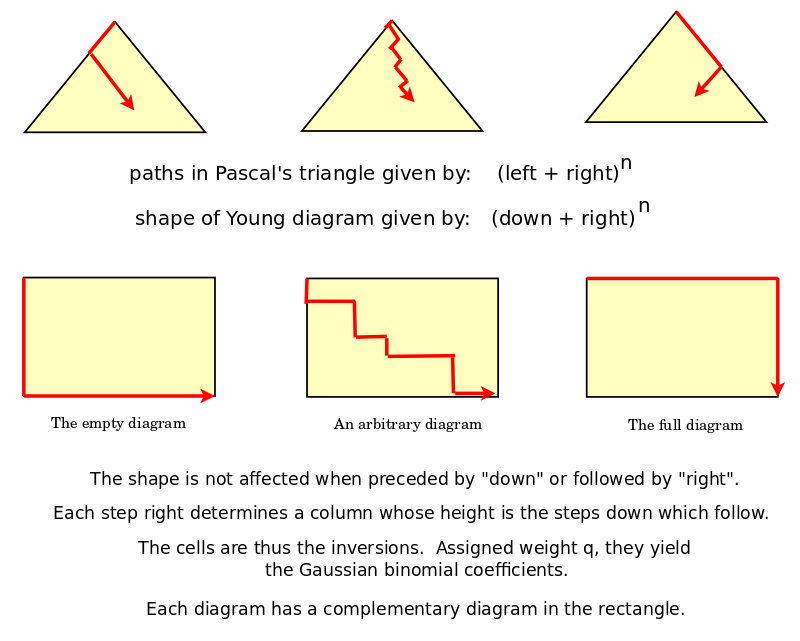• ms@ms.lt
• +370 607 27 665
• My work is in the Public Domain for all to share freely.

Introduction E9F5FC

Understandable FFFFFF

Questions FFFFC0

Notes EEEEEE

Software

Solved: Identify Young diagrams as paths in Pascal's triangle.

Each location (n i) in Pascal's triangle can be identified with the Young diagrams that fit in a rectangle with sides of length i and n-i.

Thus a Young diagram can be identified with a smallest location (n i) and all locations (m j) for which j>=i and m-j >= n-i, which is to say, for all in the triangle below (n i).Discussion

Note that each cell in the rectangle gives the choice, across terms, of x-choice-left vs. y-choice-right. So we can thus infer choices - how we would have chosen - given the choices available, and given the choices made. Inversions are related to a change in choice, in preference, and thus they provide information about changes in the chooser. Extrinsic vs. intrinsic symmetry issues become relevant.

#### YoungDiagramsAsPaths

Naujausi pakeitimai

 Puslapis paskutinį kartą pakeistas 2018 spalio 04 d., 09:38